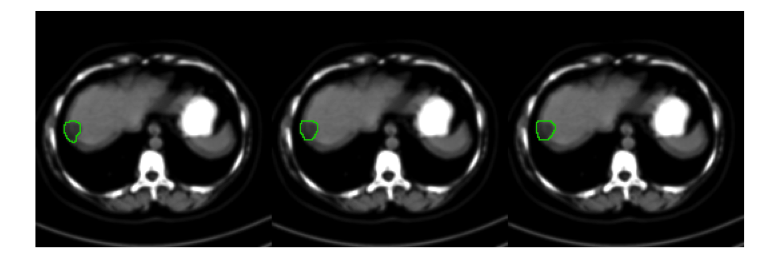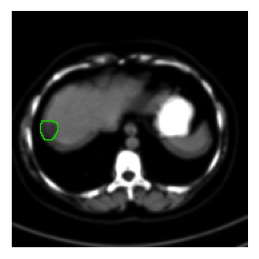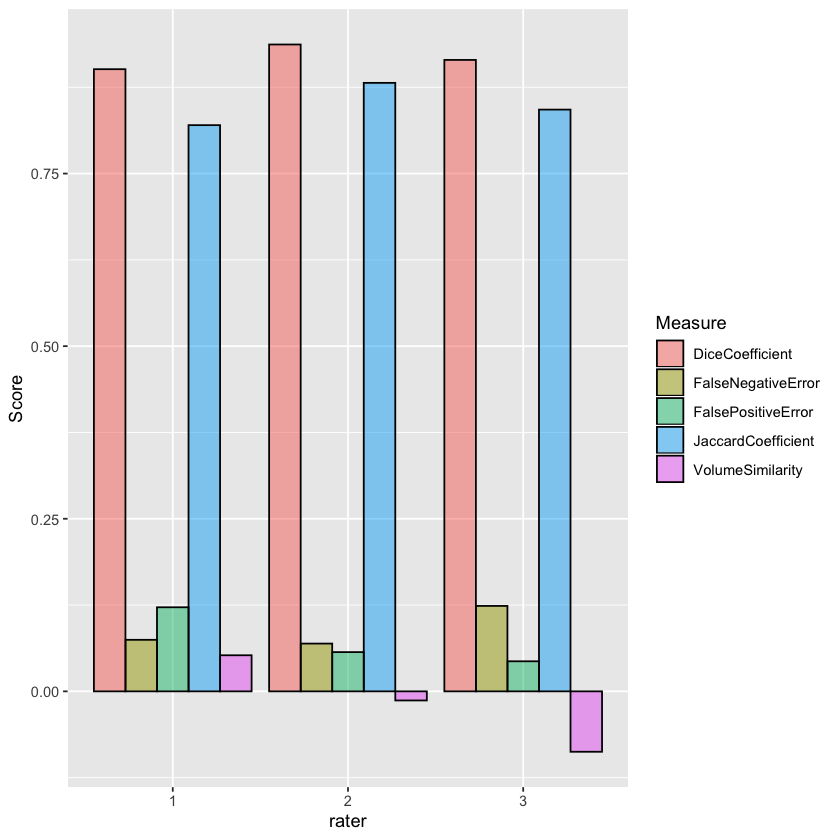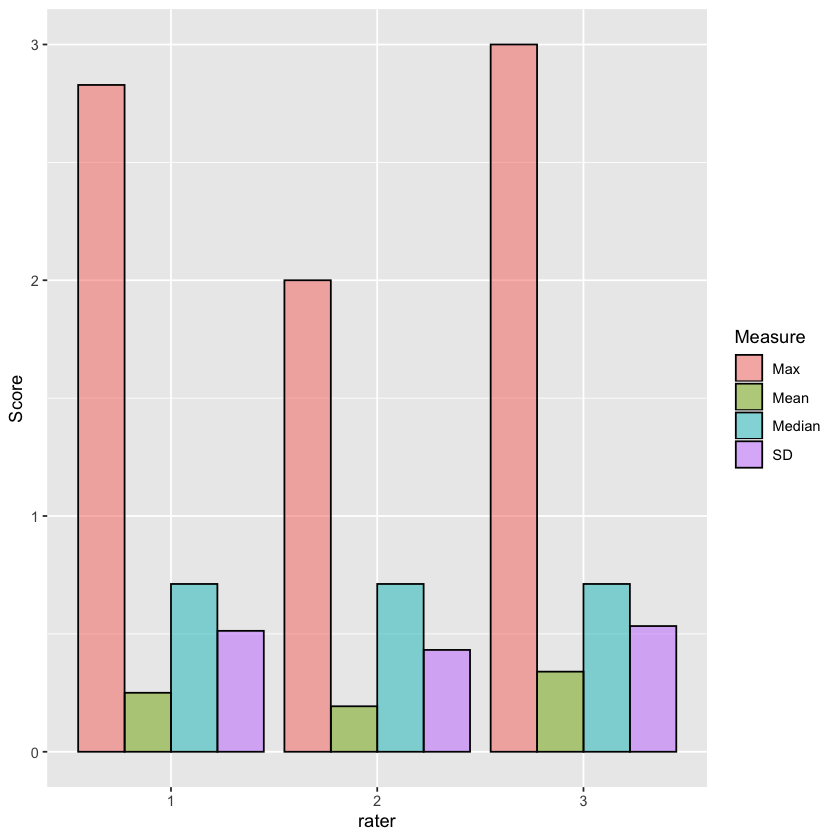# Segmentation Evaluation

Evaluating segmentation algorithms is most often done using reference data to which you compare your results.

In the medical domain reference data is commonly obtained via manual segmentation by an expert (don't forget to thank your clinical colleagues for their hard work). When you are resource limited, the reference data may be defined by a single expert. This is less than ideal. When multiple experts provide you with their input then you can potentially combine them to obtain reference data that is closer to the ever elusive "ground truth". In this notebook we show two approaches to combining input from multiple observers, majority vote and the Simultaneous Truth and Performance Level Estimation (STAPLE).

Once we have a reference, we compare the algorithm's performance using multiple criteria, as usually there is no single evaluation measure that conveys all of the relevant information. In this notebook we illustrate the use of the following evaluation criteria:

• Overlap measures:
• Jaccard and Dice coefficients
• false negative and false positive errors
• Surface distance measures:
• mean, median, max and standard deviation between surfaces
• Volume measures:
• volume similarity $\frac{2*(v1-v2)}{v1+v2}$

The relevant criteria are task dependent, so you need to ask yourself whether you are interested in detecting spurious errors or not (mean or max surface distance), whether over/under segmentation should be differentiated (volume similarity and Dice or just Dice), and what is the ratio between acceptable errors and the size of the segmented object (Dice coefficient may be too sensitive to small errors when the segmented object is small and not sensitive enough to large errors when the segmented object is large).

The data we use in the notebook is a set of manually segmented liver tumors from a single clinical CT scan. A larger dataset (four scans) is freely available from this MIDAS repository. The relevant publication is: T. Popa et al., "Tumor Volume Measurement and Volume Measurement Comparison Plug-ins for VolView Using ITK", SPIE Medical Imaging: Visualization, Image-Guided Procedures, and Display, 2006.

Note: The approach described here can also be used to evaluate Registration, as illustrated in the free form deformation notebook.

In :
library(SimpleITK)


Loading required package: rPython



## Utility functions¶

Display related utility functions.

In :
## save the default options in case you need to reset them
if (!exists("default.options"))
{
default.options <- options()
}
# display 2D images inside the notebook (colour and greyscale)
show_inline <- function(object, Dwidth=grid::unit(5, "cm"))
{
ncomp <- object$GetNumberOfComponents() if (ncomp == 3) { ## colour a <- as.array(object) a <- aperm(a, c(2, 1, 3)) } else if (ncomp == 1) { a <- t(as.array(object)) } else { stop("Only deals with 1 or 3 component images") } rg <- range(a) A <- (a - rg) / (rg - rg) dd <- dim(a) sp <- object$GetSpacing()
sz <- object$GetSize() worlddim <- sp * sz worlddim <- worlddim / worlddim W <- Dwidth H <- Dwidth * worlddim WW <- grid::convertX(W*1.1, "inches", valueOnly=TRUE) HH <- grid::convertY(H*1.1, "inches", valueOnly=TRUE) ## here we set the display size ## Jupyter only honours the last setting for a cell, so ## we can't reset the original options. That needs to ## be done manually, using the "default.options" stored above ## Obvious point to do this is before plotting graphs options(repr.plot.width = WW, repr.plot.height = HH) grid::grid.raster(A, default.units="mm", width=W, height=H) } # Tile images to create a single wider image. color_tile <- function(images) { width <- images[]$GetWidth()
height <- images[]$GetHeight() tiled_image <- Image(c(length(images) * width, height), images[]$GetPixelID(), images[]$GetNumberOfComponentsPerPixel()) for(i in 1:length(images)) { tiled_image <- Paste(tiled_image, images[[i]], images[[i]]$GetSize(), c(0, 0), c((i - 1) * width, 0))
}
return( tiled_image )
}


## Fetch the data¶

Retrieve a single CT scan and three manual delineations of a liver tumor. Visual inspection of the data highlights the variability between experts.

All computations are done in 3D (the dimensionality of the images). For display purposes we selected a single slice_for_display. Change this variable's value to see other slices.

In :
slice_for_display <- 77

# For display we need to window-level the slice (map the high dynamic range to a reasonable display)
display_slice <- Cast(IntensityWindowing(image[,,slice_for_display],
windowMinimum=-1024,
windowMaximum=976),
"sitkUInt8")

# Overlay the segmentation contour from each of the segmentations onto the "slice_for_display"
display_overlays <- lapply(segmentations,
function(seg) LabelMapContourOverlay(Cast(seg[,,slice_for_display], "sitkLabelUInt8"),
display_slice,
opacity = 1))

show_inline(color_tile(display_overlays),grid::unit(15, "cm"))## Derive a reference¶

There are a variety of ways to derive a reference segmentation from multiple expert inputs. Several options, there are more, are described in "A comparison of ground truth estimation methods", A. M. Biancardi, A. C. Jirapatnakul, A. P. Reeves.

Two methods that are available in SimpleITK are majority vote and the STAPLE algorithm.

In :
# Use majority voting to obtain the reference segmentation. Note that this filter does not resolve ties. In case of
# ties, it will assign max_label_value+1 or a user specified label value (labelForUndecidedPixels) to the result.
# Before using the results of this filter you will have to check whether there were ties and modify the results to
# resolve the ties in a manner that makes sense for your task. The filter implicitly accommodates multiple labels.
labelForUndecidedPixels <- 10
reference_segmentation_majority_vote <- LabelVoting(segmentations, labelForUndecidedPixels)

show_inline(LabelMapContourOverlay(Cast(reference_segmentation_majority_vote[,,slice_for_display], "sitkLabelUInt8"), display_slice, opacity = 1),
grid::unit(5, "cm"))In :
# Use the STAPLE algorithm to obtain the reference segmentation. This implementation of the original algorithm
# combines a single label from multiple segmentations, the label is user specified. The result of the
# filter is the voxel's probability of belonging to the foreground. We then have to threshold the result to obtain
# a reference binary segmentation.
foregroundValue <- 1
threshold <- 0.95
reference_segmentation_STAPLE_probabilities <- STAPLE(segmentations, foregroundValue)
# We use the overloaded operator to perform thresholding, another option is to use the BinaryThreshold function.
reference_segmentation_STAPLE <- reference_segmentation_STAPLE_probabilities > threshold

show_inline(LabelMapContourOverlay(Cast(reference_segmentation_STAPLE[,,slice_for_display], "sitkLabelUInt8"), display_slice, opacity = 1),
grid::unit(5, "cm"))## Evaluate segmentations using the reference¶

Once we derive a reference from our experts input we can compare segmentation results to it.

Note that in this notebook we compare the expert segmentations to the reference derived from them. This is not relevant for algorithm evaluation, but it can potentially be used to rank your experts.

### Utility functions¶

These functions compute standard overlap and surface distance measures used when comparing segmentations.

In :
# Compare the two given segmentations using overlap measures (Jaccard, Dice, etc.)
compute_overlap_measures <- function(segmentation, reference_segmentation)
{
omf <- LabelOverlapMeasuresImageFilter()
omf$Execute(reference_segmentation, segmentation) result <- c(omf$GetJaccardCoefficient(), omf$GetDiceCoefficient(), omf$GetVolumeSimilarity(), omf$GetFalseNegativeError(), omf$GetFalsePositiveError())
names(result) <- c("JaccardCoefficient", "DiceCoefficient", "VolumeSimilarity",
"FalseNegativeError", "FalsePositiveError")
return (result)
}

# Compare a segmentation to the reference segmentation using distances between the two surfaces. To facilitate
# surface distance computations we use a distance map of the reference segmentation.
compute_surface_distance_measures <- function(segmentation, reference_distance_map)
{
segmented_label = 1

# Get the intensity statistics associated with each of the labels, combined
# with the distance map image this gives us the distances between surfaces.
lisf <- LabelIntensityStatisticsImageFilter()

# Get the pixels on the border of the segmented object
segmented_surface <- LabelContour(segmentation)
lisf$Execute(segmented_surface, reference_distance_map) result <- c(lisf$GetMean(segmented_label), lisf$GetMedian(segmented_label), lisf$GetStandardDeviation(segmented_label), lisf$GetMaximum(segmented_label)) names(result) <- c("Mean", "Median", "SD", "Max") return (result) }  Evaluate the three segmentations with respect to the STAPLE based reference. In : overlap_measures <- t(sapply(segmentations, compute_overlap_measures, reference_segmentation=reference_segmentation_STAPLE)) overlap_measures <- as.data.frame(overlap_measures) overlap_measures$rater <- rownames(overlap_measures)

distance_map_filter <- SignedMaurerDistanceMapImageFilter()
distance_map_filter$SquaredDistanceOff() STAPLE_reference_distance_map <- abs(distance_map_filter$Execute(reference_segmentation_STAPLE))

surface_distance_measures <- t(sapply(segmentations,
compute_surface_distance_measures,
reference_distance_map=STAPLE_reference_distance_map))
surface_distance_measures <- as.data.frame(surface_distance_measures)
surface_distance_measures$rater <- rownames(surface_distance_measures) # Look at the results using the notebook's default display format for data frames overlap_measures surface_distance_measures  A data.frame: 3 × 6 JaccardCoefficientDiceCoefficientVolumeSimilarityFalseNegativeErrorFalsePositiveErrorrater <dbl><dbl><dbl><dbl><dbl><chr> 0.82012410.9011738 0.052252930.074650080.121771221 0.88137930.9369501-0.013196480.069191550.056826572 0.84265280.9146083-0.087508820.123732250.043542443 A data.frame: 3 × 5 MeanMedianSDMaxrater <dbl><dbl><dbl><dbl><chr> 0.25020100.71177410.51293762.8284271 0.19294640.71177410.43198932.0000002 0.33983960.71177410.53320393.0000003 ## Improved output¶ If the tidyr and ggplot2 packages are installed in your R environment then you can easily produce high quality output. In : library(tidyr) library(ggplot2) ## reset the plot size options(default.options) overlap.gathered <- gather(overlap_measures, key=Measure, value=Score, -rater) ggplot(overlap.gathered, aes(x=rater, y=Score, group=Measure, fill=Measure)) + geom_bar(stat="identity", position="dodge", colour='black', alpha=0.5) surface_distance.gathered <- gather(surface_distance_measures, key=Measure, value=Score, -rater) ggplot(surface_distance.gathered, aes(x=rater, y=Score, group=Measure, fill=Measure)) + geom_bar(stat="identity", position="dodge", colour='black', alpha=0.5)You can also export the data as a table for your LaTeX manuscript using the xtable package, just copy paste the output of the following cell into your document. In : library(xtable) sd <- surface_distance_measures sd$rater <- NULL
print(xtable(sd, caption="Segmentation surface distance measures per rater.",
label="tab:surfdist", digits=2))

% latex table generated in R 3.5.3 by xtable 1.8-4 package
% Thu Jan 30 13:51:51 2020
\begin{table}[ht]
\centering
\begin{tabular}{rrrrr}
\hline
& Mean & Median & SD & Max \\
\hline
1 & 0.25 & 0.71 & 0.51 & 2.83 \\
2 & 0.19 & 0.71 & 0.43 & 2.00 \\
3 & 0.34 & 0.71 & 0.53 & 3.00 \\
\hline
\end{tabular}
\caption{Segmentation surface distance measures per rater.}
\label{tab:surfdist}
\end{table}